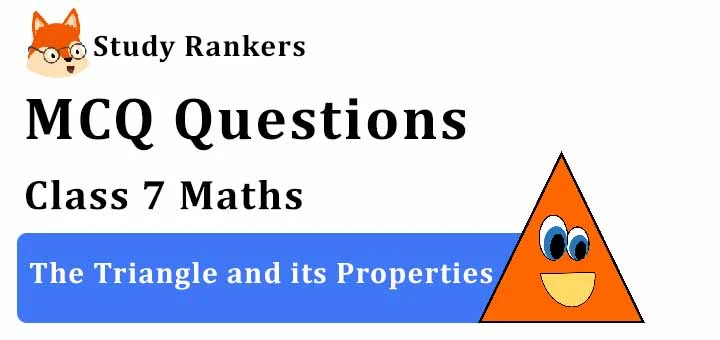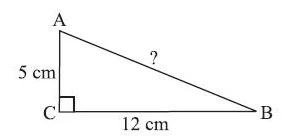## MCQ Questions for Class 7 Maths: Ch 6 The Triangle and its Properties1. A triangle in which two sides are of equal lengths is called _______________.
(a) scalene
(b) acute-angled
(c) equilateral
(d) isosceles
► (d) isosceles

2. ABC is an isosceles triangle with AB = AC and AD is altitude, then ____.
(a) ∠B>∠C
(b) ∠B<∠C
(c) ∠B=∠C
(d) None of these
► (c) ∠B=∠C

3. The triangle whose lengths of sides are 3 cm, 4 cm, 5 cm is a
(a) obtuse-angled triangle
(b) right-angled triangle
(c) acute-angled triangle
(d) None of these
► (b) right-angled triangle

4. ΔABC is right-angled at C. If AC = 5 cm and BC = 12 cm find the length of AB.(a) 13 cm
(b) 11 cm
(c) 20 cm
(d) None of these
► (a) 13 cm

5. The sum of the lengths of any two sides of a triangle is _____________ the third side of the triangle.
(a) greater than
(b) half
(c) less than
(d) double
► (a) greater than

6. A/an _____________ connect a vertex of a triangle to the mid-point of the opposite side.
(a) altitude
(b) median
(c) vertex
(d) none of these
► (b) median

7. How many medians a triangle can have?
(a) 1
(b) 2
(c) 3
(d) none of these
► (c) 3

8. Which is the longest side of a right triangle?
(a) Base
(b) Perpendicular
(c) Hypotenuse
(d) None of these
► (c) Hypotenuse

9. How many altitudes can a triangle have?
(a) 1
(b) 2
(c) 3
(d) None of these
► (c) 3

10. Which is the longest side in the triangle ABC right angled at B?
(a) AB
(b) AC
(c) BC
(d) None of these
► (b) AC

11. If the angles of a triangle are in the ratio 4:5:9. Find all the angles of a the triangle
(a) 40 deg, 50 deg , 90 deg
(b) 90 deg, 72 deg, 18 deg
(c) 9 deg, 90 deg, 55 deg
(d) 45 deg, 60 deg, 18 deg
► (a) 40 deg, 50 deg , 90 deg

12. One of the angles of a triangle is 110° and the other two angles are equal what is the measure of each of these equal angles
(a) 35°,35°
(b) 40° , 40°
(c) 11°, 11°
(d) 80° , 80°
► (a) 35°,35°

13. Which is the longest side in the triangle PQR right angled at P?
(a) PQ
(b) QR
(c) PR
(d) None of these
► (b) QR

14. In a ΔABC,which of the given condition holds?
(a) AB−BC>CA
(b) AB+BC<CA
(c) AB−BC<CA
(d) AB+CA<BC
► (c) AB−BC<CA

15. In the Pythagoras property, the triangle must be ___________ .
(a) obtuse-angled
(b) acute-angled
(c) right-angled
(d) none of these
► (c) right-angled

16. ΔPQR is a triangle right-angled at P. If PQ = 3 cm and PR = 4 cm, find QR.
(a) 8 cm
(b) 5 cm
(c) 7 cm
(d) 3 cm
► (b) 5 cm

17. In a ΔABC,if AB+BC = 10 cm, BC+CA = 12 cm,CA+AB = 16 cm, then the perimeter of the triangle is ____.
(a) 19cm
(b) 17cm
(c) 28cm
(d) 22cm
► (a) 19cm

18. Aryan wants to plant a flower on the ground in the form of a rhombus. The diagonals of the rhombus measures 42 cm and 56 cm. Find the perimeter of the field.
(a) 150 cm
(b) 140 cm
(c) 130cm
(d) 120cm
► (b) 140 cm